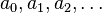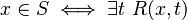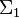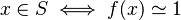# User:IssaRice/Computability and logic/Characterization of recursively enumerable sets

Let$S$ be a set of natural numbers. The following are all equivalent.

Property Emphasis
The elements of$S$ can be enumerated in a computable manner as$a_0, a_1, a_2, \ldots$ Recursively enumerable$S$ is the range of a computable partial function. Recursively enumerable$S$ is empty or the range of a computable total function. Recursively enumerable$S$ is empty or the range of a primitive recursive function. Recursively enumerable$S$ is the domain of a computable partial function. Semi-ness
A recursive semicharacteristic function for$S$ exists. Semi-ness
There exists a two-place recursive relation$R$ such that$x \in S \iff \exists t\ R(x,t)$ Semi-ness
The relation$x \in S$ is$\Sigma_1$, i.e., there exists a$(k+1)$-place recursive relation$R$ such that$x \in S \iff \exists t_1 \cdots \exists t_k\ R(x,t_1,\ldots,t_k)$ Semi-ness
The relation$x\in S$ has a computable partial verifying function, i.e., there exists a computable partial function$f$ such that$x \in S \iff f(x) \simeq 1$. Semi-ness

The rows labeled "Recursively enumerable" all emphasize the fact that$S$ is "recursively enumerable", i.e., that the elements of$S$ can be listed in a recursive (computable) way.

The rows labeled "Semi-ness" all emphasize the fact that$S$ is semidecidable/recognizable/verifiable/semirecursive, i.e., that if something is in$S$, then in a finite amount of time we can verify this computably, but that if something isn't in$S$, then we will run into an infinite loop.

The fundamental theorem of recursively enumerable sets says that recursively enumerable equals semi-ness.